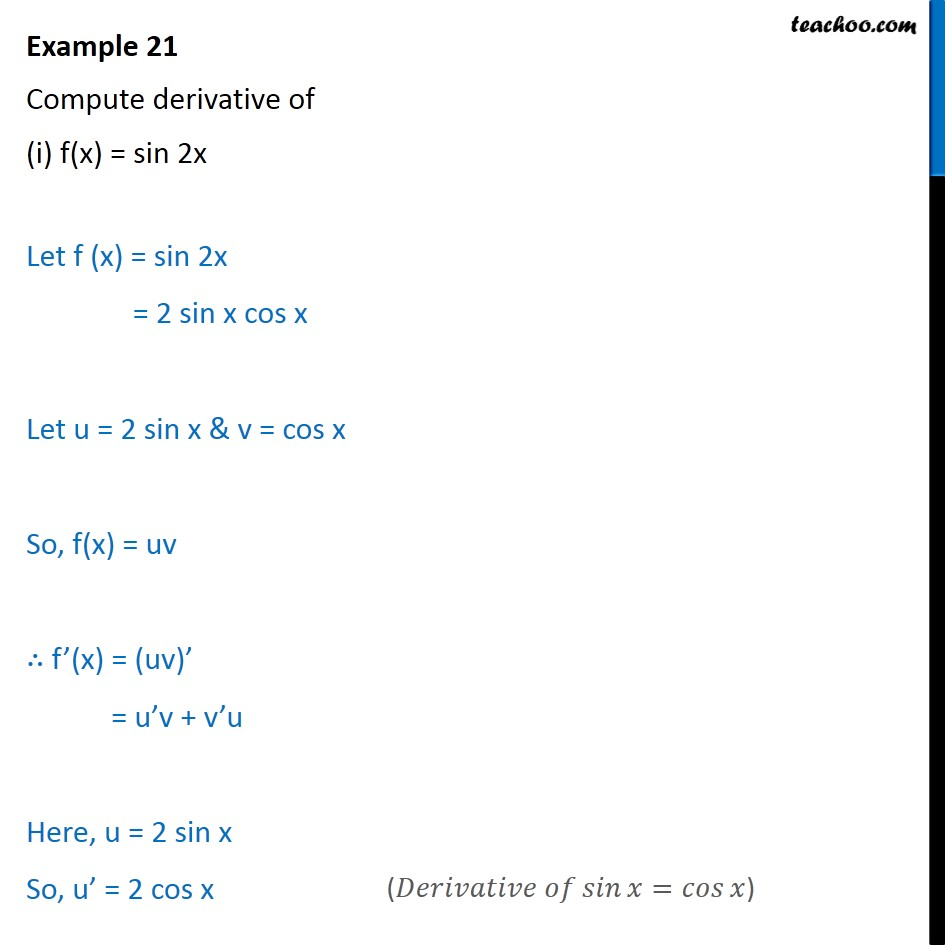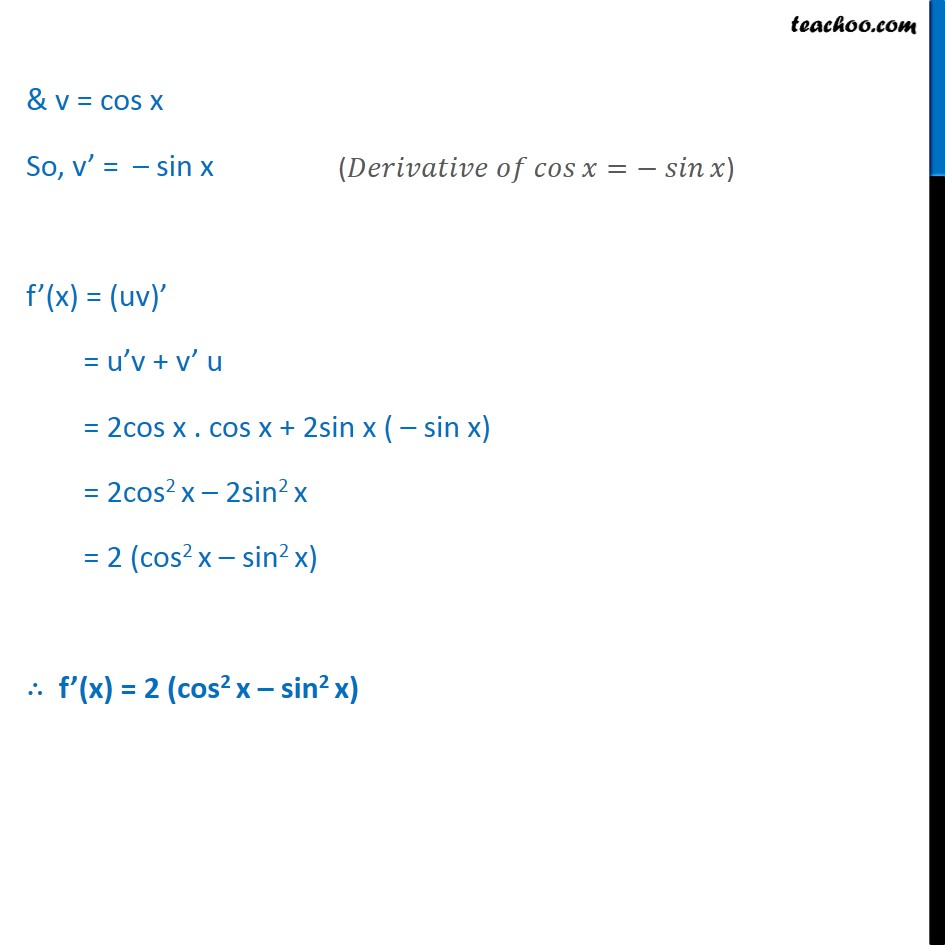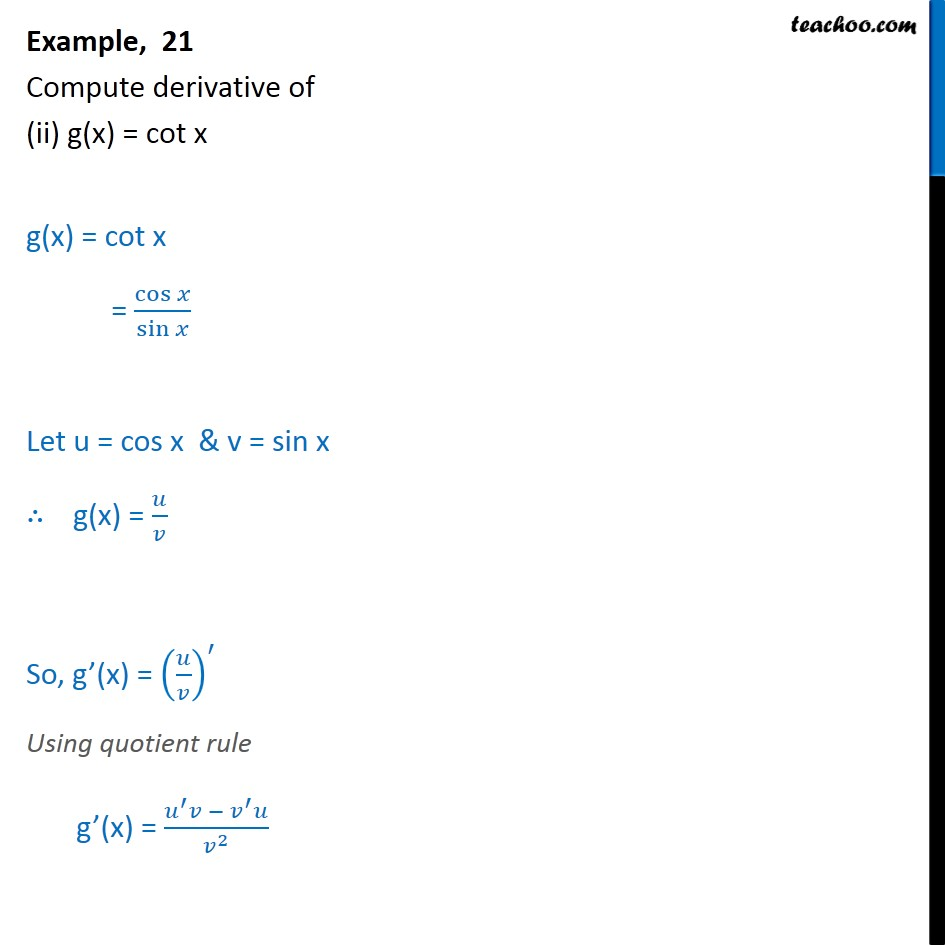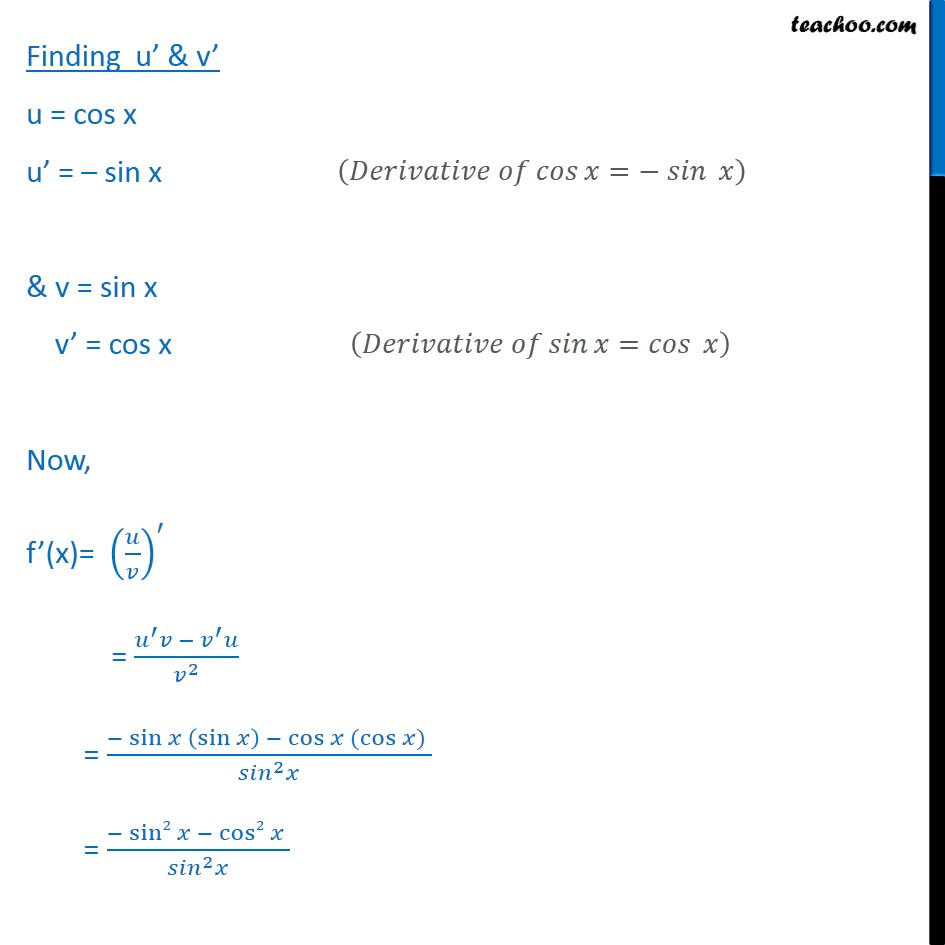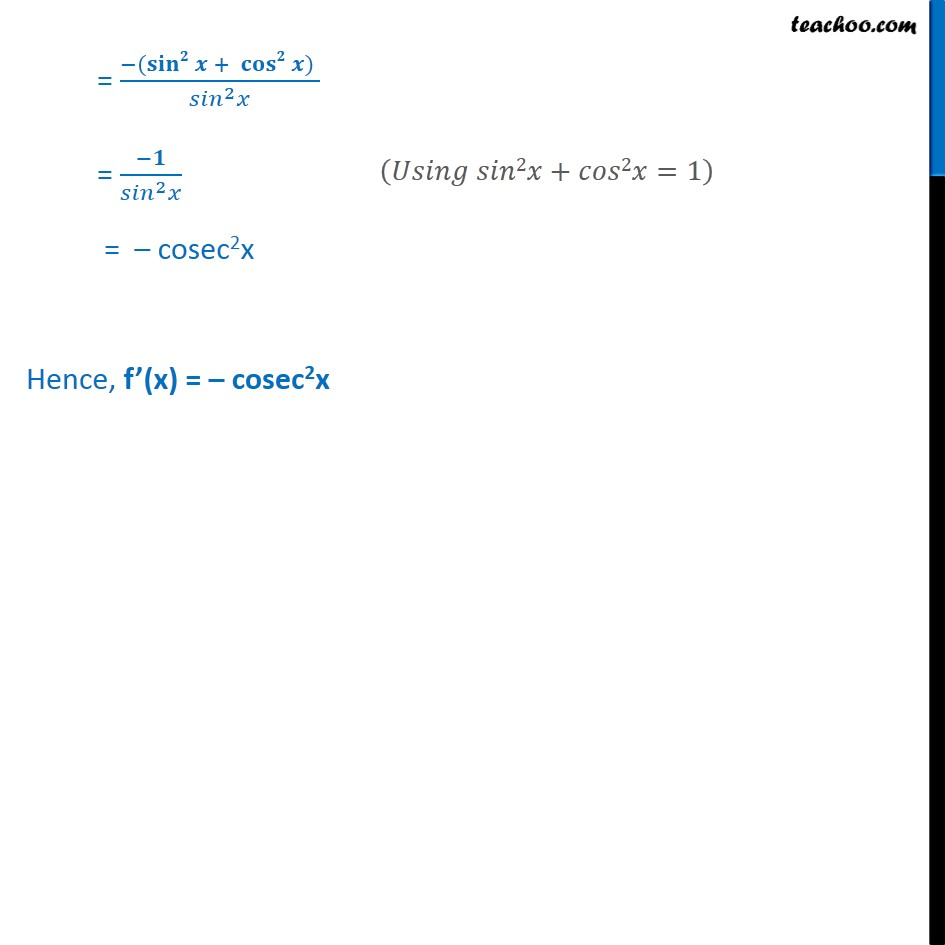1. Chapter 13 Class 11 Limits and Derivatives
2. Serial order wise
3. Examples

Transcript

Example 21 Compute derivative of (i) f(x) = sin 2x Let f (x) = sin 2x = 2 sin x cos x Let u = 2 sin x & v = cos x So, f(x) = uv ∴ f’(x) = (uv)’ = u’v + v’u Here, u = 2 sin x So, u’ = 2 cos x & v = cos x So, v’ = – sin x f’(x) = (uv)’ = u’v + v’ u = 2cos x . cos x + 2sin x ( – sin x) = 2cos2 x – 2sin2 x = 2 (cos2 x – sin2 x) = 2 cos 2x ∴ f’(x) = 2 cos 2x Example, 21 Compute derivative of (ii) g(x) = cot x g(x) = cot x = cos﷮𝑥﷯﷮ sin﷮𝑥﷯﷯ Let u = cos x & v = sin x ∴ g(x) = 𝑢﷮𝑣﷯ So, g’(x) = 𝑢﷮𝑣﷯﷯﷮′﷯ Using quotient rule g’(x) = 𝑢﷮′﷯𝑣 − 𝑣﷮′﷯𝑢﷮ 𝑣﷮2﷯﷯ Finding u’ & v’ u = cos x u’ = – sin x & v = sin x v’ = cos x Now, f’(x)= 𝑢﷮𝑣﷯﷯﷮′﷯ = 𝑢﷮′﷯𝑣 − 𝑣﷮′﷯𝑢﷮ 𝑣﷮2﷯﷯ = − sin﷮𝑥 sin﷮𝑥﷯﷯ − cos﷮𝑥 ( cos﷮𝑥)﷯ ﷯﷯﷮ 𝑠𝑖𝑛﷮2﷯𝑥﷯ = − sin2﷮𝑥 − cos2﷮𝑥 ﷯﷯﷮ 𝑠𝑖𝑛﷮2﷯𝑥﷯ = −( 𝐬𝐢𝐧𝟐﷮𝒙 + 𝐜𝐨𝐬𝟐﷮𝒙) ﷯﷯﷮ 𝑠𝑖𝑛﷮2﷯𝑥﷯ = −𝟏﷮ 𝑠𝑖𝑛﷮2﷯𝑥﷯ = – cosec2x Hence, f’(x) = – cosec2x

Examples